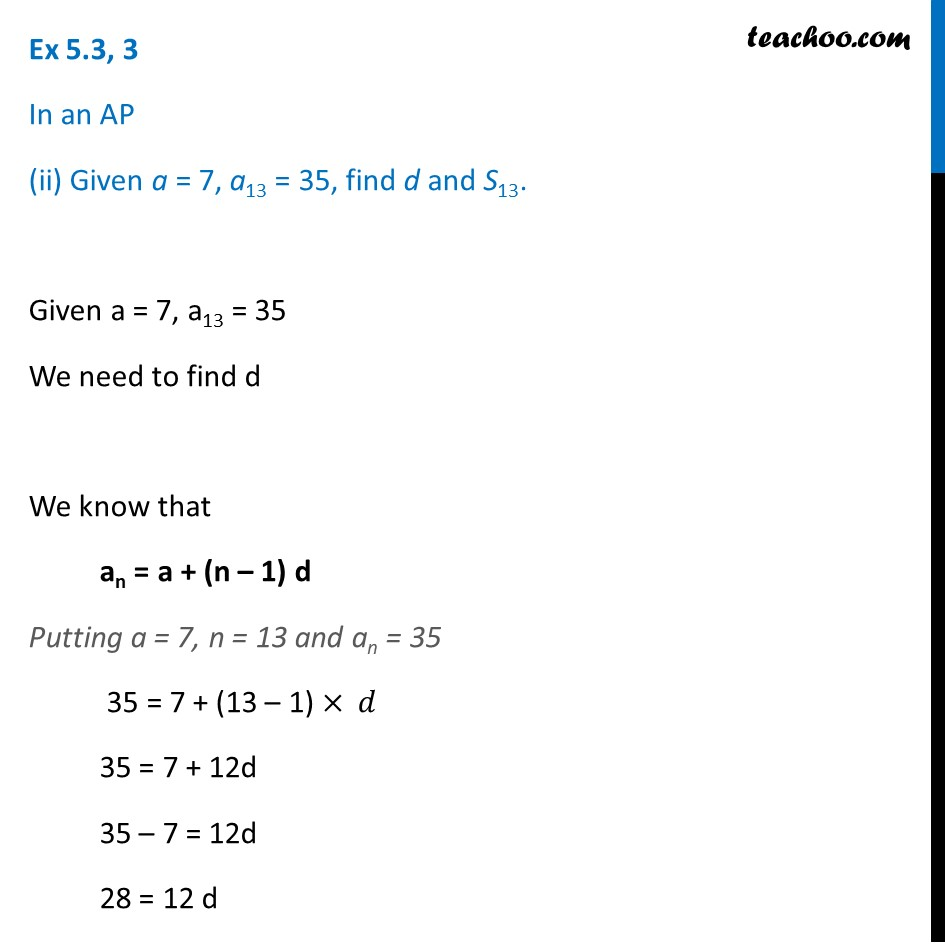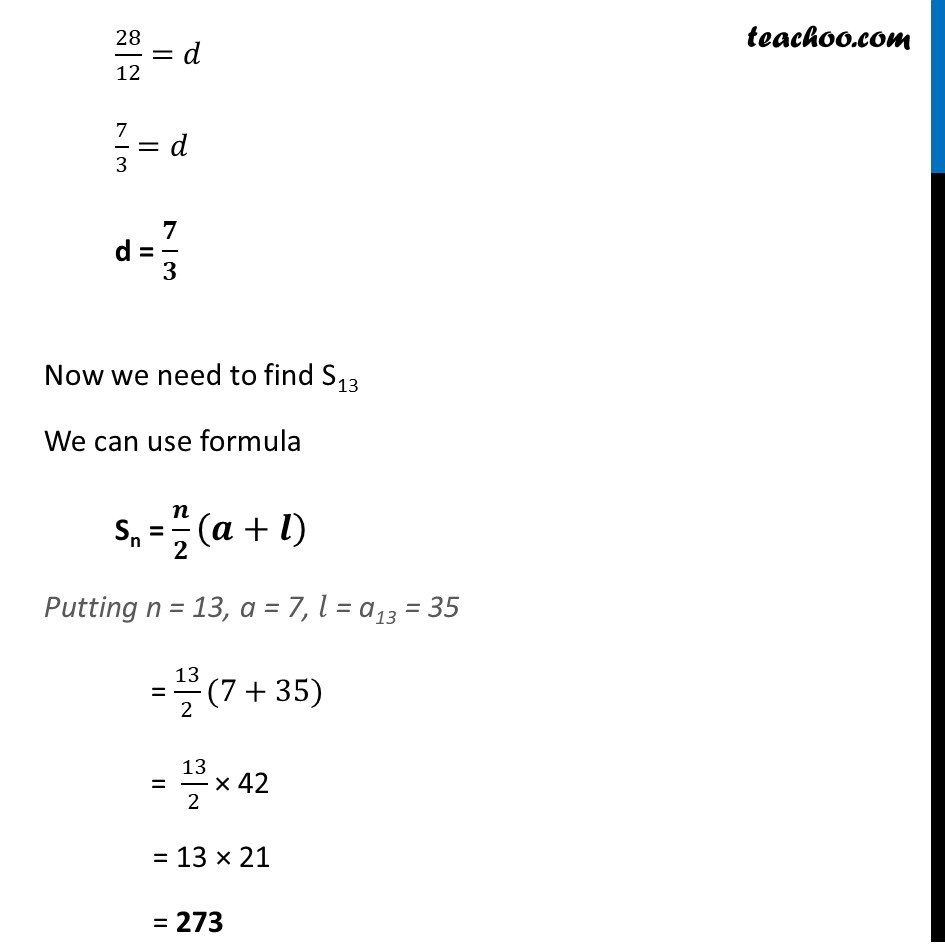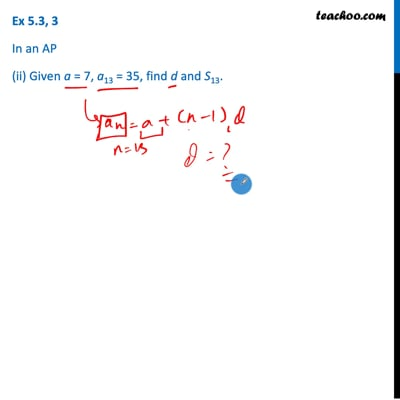Ex 5.3

Chapter 5 Class 10 Arithmetic Progressions (Term 2)
Serial order wiseThis video is only available for Teachoo black users

### Transcript

Ex 5.3, 3 In an AP (ii) Given a = 7, a13 = 35, find d and S13. Given a = 7, a13 = 35 We need to find d We know that an = a + (n – 1) d Putting a = 7, n = 13 and an = 35 35 = 7 + (13 – 1) × 𝑑 35 = 7 + 12d 35 – 7 = 12d 28 = 12 d 28/12=𝑑 7/3=𝑑 d = 𝟕/𝟑 Now we need to find S13 We can use formula Sn = 𝒏/𝟐 (𝒂+𝒍) Putting n = 13, a = 7, 𝑙 = a13 = 35 = 13/2(7+35) = 13/2 × 42 = 13 × 21 = 273